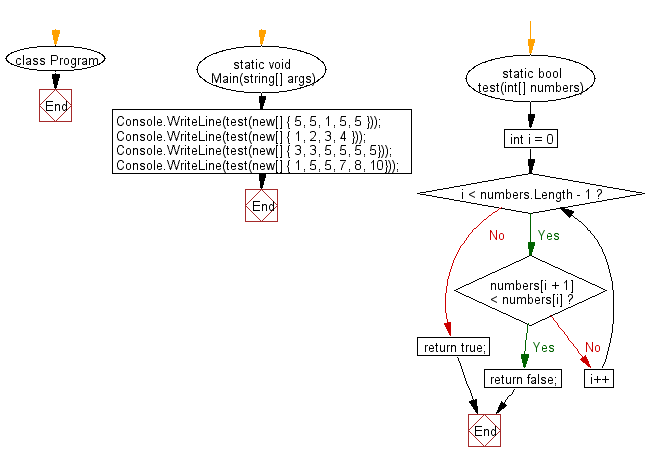﻿ C# - Each value in an array is larger, equal to the previous# C# Sharp Basic Algorithm Exercises: Check whether the value of each element is equal or greater than the value of previous element of a given array of integers

## C# Sharp Basic Algorithm: Exercise-133 with Solution

Write a C# Sharp program to check whether the value of each element is equal or greater than the value of previous element of a given array of integers.

Sample Solution:

C# Sharp Code:

``````using System;
namespace exercises
{
class Program
{
static void Main(string[] args)
{
Console.WriteLine(test(new[] { 5, 5, 1, 5, 5 }));
Console.WriteLine(test(new[] { 1, 2, 3, 4 }));
Console.WriteLine(test(new[] { 3, 3, 5, 5, 5, 5}));
Console.WriteLine(test(new[] { 1, 5, 5, 7, 8, 10}));
}
static bool test(int[] numbers)
{
for (int i = 0; i < numbers.Length - 1; i++)
{
if (numbers[i + 1] < numbers[i]) return false;
}

return true;
}

}
}
```
```

Sample Output:

```False
True
True
True
```

Flowchart:C# Sharp Code Editor:

Improve this sample solution and post your code through Disqus

What is the difficulty level of this exercise?

Test your Programming skills with w3resource's quiz.

﻿